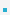1. Basics of Structures

A structure is a collection of related variables (of possibly different types) grouped together under a single name. This is a an example of composition–building complex structures out of simple ones.

Examples
 struct point {     int x;     int y; }; // notice the ; at the end
 struct student {     char name;     int id;     int age; };A struct declaration defines a type.The name of the structure is optional
struct { ... } x, y, z;The variables declared within a structure are called its members.Variables can be declared like any other built in data-type.
struct point pt;Initialization is done by specifying values of every member.
struct point pt={10, 20};Assignment operator copies every member of the structure (be careful with pointers).Members can be structures
 struct triangle { struct point ptA; struct point ptB; struct point ptC; };Members can be self referential
 struct element {     int data;     struct element *next; }Individual members can be accessed using ‘.’ operator.
 struct point pt={10, 20}; int x=pt.x; int y=pt.y;If structure is nested, multiple ‘.’ are required
 struct rectangle {     struct point tl; /∗ top left ∗/     struct point br; /∗ bottom right ∗/ }; struct rectangle rect ; int tlx= rect.tl.x; /∗ nested ∗/ int tly= rect.tl.y;

 이전페이지 / 2 / 다음페이지